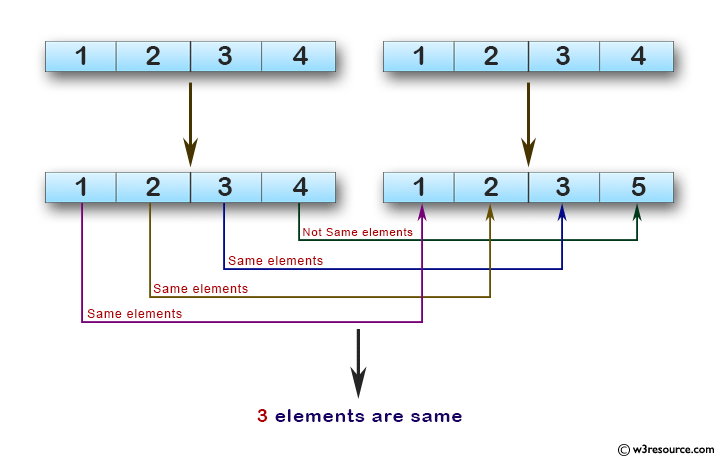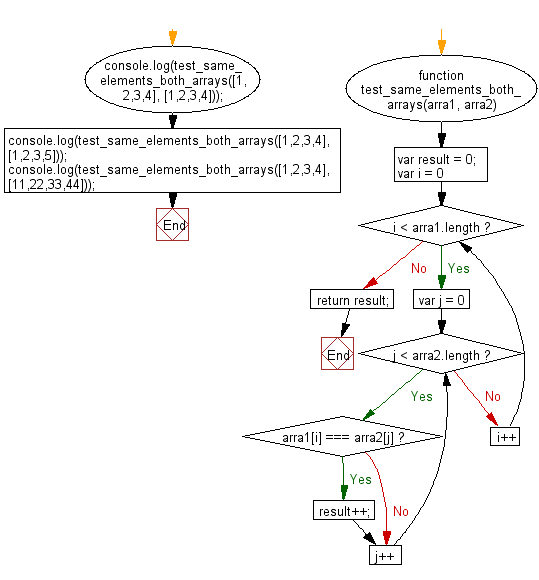# JavaScript: Find the number of elements which presents in both of the given arrays

## JavaScript Basic: Exercise-141 with Solution

Write a JavaScript program to find the number of elements which presents in both of the given arrays.

Pictorial Presentation:Sample Solution:

HTML Code:

``````<!DOCTYPE html>
<html>
<meta charset="utf-8">
<meta name="viewport" content="width=device-width">
<title>Find the number of elements which presents in both of the given arrays.</title>
<body>

</body>
</html>
```
```

JavaScript Code:

``````function test_same_elements_both_arrays(arra1, arra2) {
var result = 0;
for(var i = 0; i < arra1.length; i++) {
for(var j = 0; j < arra2.length; j++){
if(arra1[i] === arra2[j])
{
result++;
}
}
}
return result;
}
console.log(test_same_elements_both_arrays([1,2,3,4], [1,2,3,4]));
console.log(test_same_elements_both_arrays([1,2,3,4], [1,2,3,5]));
console.log(test_same_elements_both_arrays([1,2,3,4], [11,22,33,44]));
``````

Sample Output:

```4
3
0
```

Flowchart:ES6 Version:

``````function test_same_elements_both_arrays(arra1, arra2) {
let result = 0;
for(let i = 0; i < arra1.length; i++) {
for(let j = 0; j < arra2.length; j++){
if(arra1[i] === arra2[j])
{
result++;
}
}
}
return result;
}
console.log(test_same_elements_both_arrays([1,2,3,4], [1,2,3,4]));
console.log(test_same_elements_both_arrays([1,2,3,4], [1,2,3,5]));
console.log(test_same_elements_both_arrays([1,2,3,4], [11,22,33,44]));
``````

Live Demo:

See the Pen javascript-basic-exercise-141 by w3resource (@w3resource) on CodePen.

Improve this sample solution and post your code through Disqus

What is the difficulty level of this exercise?

Test your Programming skills with w3resource's quiz.

﻿

## JavaScript: Tips of the Day

Checks if a string is an anagram of another string (case-insensitive, ignores spaces, punctuation and special characters)

Example:

```const isAnagram = (str1, str2) => {
const normalize = str =>
str
.toLowerCase()
.replace(/[^a-z0-9]/gi, '')
.split('')
.sort()
.join('');
return normalize(str1) === normalize(str2);
};
console.log(isAnagram('iceman', 'cinema')); // true
```

Output:

```true
```